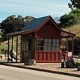# Gas station without pumps

## 2017 July 9

### Digital tone detection with Goertzel’s algorithm

Filed under: Robotics,Uncategorized — gasstationwithoutpumps @ 00:28
Tags: , ,

In Multiple-feedback bandpass active filter I built a simple active filter to be part of a tone-detection circuit (looking for 2kHz).  Although the filter worked, it did not have as much gain as I expected, its out-of-band rejection was not impressive, and centering it at 20kHz accurately would require adding a potentiometer to tune it. To make a full tone detector, I’d have to have a rectifier and low-pass filter (or some other envelope detector).

I thought that it would be simpler to do the whole thing digitally, as long as my input signal was large enough to be reasonably measured by an analog-to-digital converter.  I started designing a digital filter, with the intent of implementing the same block diagram: bandpass filter, rectifier, and low-pass filter.  Although I came up with a fairly simple filter that looked like it would work well, my son suggested a different approach.  If I don’t need the tone detection to be super-fast, I can process the data a block at a time, and use Goertzel’s algorithm to compute the power at the frequency of interest.  By playing with the block length and windowing functions, I can shape the detection to have different bandwidths and shapes, but with a far steeper rolloff than with the active filter.

Goertzel’s algorithm is a way to compute one coefficient of a discrete Fourier transform (DFT) efficiently with only real-number computation.  In fact, with judicious scaling, I was able to do the computation with just 32-bit integer arithmetic, which can be done very quickly on microcontrollers (like the K20 processor used in the Teensy3.2 boards).

The per-sample computation is just a digital filter with transfer function$\frac{S(z)}{X(z)} = \frac{1}{1 - 2 \cos(\omega_0) z^{-1} + z^{-2}} = \frac{1}{\left(1 - e^{+j \omega_0} z^{-1}\right)\left(1 - e^{-j \omega_0} z^{-1}\right)}~,$

where$\omega_0 = 2\pi f_0/f_s$ is the center frequency in radians, with$f_s$ being the sampling frequency.  This filter is not quite stable (poles right on the unit circle), so we can’t run it continuously without danger of overflow.  However, if we run it for just N samples, it essentially computes one term of the DFT (with a little post-processing magic):$y[n] = e^{j 2 \pi \frac{k}{N}} s[N-1] - s[N-2] ~.$

I chose a sampling rate of 16kHz, so that my 2kHz center frequency is$\omega_0=\pi/4$, and the coefficient for$z^{-1}$ is$\sqrt{2}$.

The algorithm I used is

sprev = 0
sprev2 = 0

for i in range(N):
x = int(round(2**15 * (1+cos(freq/fs*2*pi*i))))  # full-scale unsigned 16-bit sine wave for testing
x = x* window[i]//2**14
s = x + sprev*coeff//scale - sprev2
sprev2 = sprev
sprev = s

scaled_sp2 = sprev2//N
scaled_sp = sprev//N
scaled_power =  scaled_sp2 * scaled_sp2 + scaled_sp * scaled_sp - coeff * scaled_sp * scaled_sp2//scale


The square-root of two for the coefficient in the recurrence was represented by coeff=181 and scale=128. The block length (N) was chosen to be 160, so that a block would be processed every 10ms. A 100Hz update rate is fairly typical for systems that control mechanical devices (50Hz is often good enough), and is quick enough not to be a perceptible delay.

I also kept track of the maximum absolute value for s, to make sure that I never exceeded 32 bits anywhere in the computation.
Here is a plot of the scaled_power output, as a function of the sine-wave frequency:With a rectangular window, the detector has a bandwidth of about 89 Hz, for a Q of 22.5, but the sidelobes are pretty big.

To suppress the sidelobes, I prescaled the signals by a Slepian window, which maximizes the energy in the mainlobe. The Slepian window was computed by the SciPy signal-processing package, scaled to be full-range unsigned 16-bit integers.  The width of the mainlobe is somewhat adjustable—I chose a setting that gave me a somewhat lower Q (wider bandwidth), so that the filter could tolerate some mistuning of the center frequency in the source.The sidelobes are so small that they get rounded to 0 or 1, while 2kHz is about 384e6, a ratio of about 85dB. The half-power bandwidth is about 226Hz, for a Q of about 8.85.

I could reduce the Slepian width to 0.1, getting a bandwidth of 192Hz (Q of 10.4) and still have the sidelobes fully suppressed. Trying to get higher Q with a block length of 160 starts getting sidelobes, but increasing N allows smaller bandwidths.

I suppose that my next step is to implement this algorithm in C on a Teensy 3.2, connected to an ADC, and see how well it performs in the real world. It looks very promising.

## 1 Comment »

1.[…] I’m planning to sit in on CMPE 118/L (Mechatronics) this fall, and so I started looking over some of the material for the course from previous years.  One exercise involved designing a “beacon detector” that signals when it sees an infrared signal modulated with a 2kHz square wave. The exercise calls for an all-analog solution (phototransistor, transimpedance amplifier, active filter, rectifier, peak detector, …), which I plan to do when the time comes, but I got intrigued by the idea of doing an almost purely digital design.  That was the motivation for the Goertzel filter blog post. […]

Pingback by Beacon detector board | Gas station without pumps — 2017 August 6 @ 18:27

This site uses Akismet to reduce spam. Learn how your comment data is processed.